# Equation of tangent

## Equation of Tangent

(a) At (x1, y1)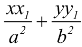= 1

### (b) At (acosθ, bsinθ )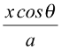+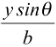= 1

### (c) In slope form

y = mx ±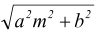Equation of Normal

(a) At (x1, y1) is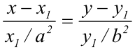(b) At (acosθ, bsinθ ) is axsecθ − bycosecθ = a2 − b2